# Controller design and stability analysis of a respiratory ventilator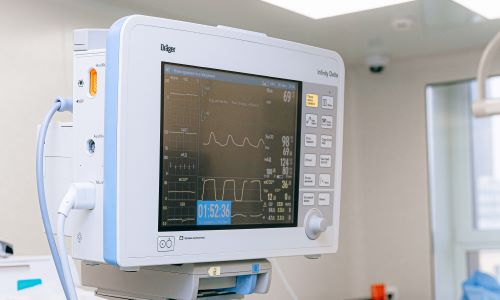Patients with respiratory distress, for example breathing difficulty due to a viral infection, often require breathing assistance through machines. This is known as mechanical ventilation or assisted ventilation. Machines can assist in breathing through two ways:

• Positive pressure ventilation: This is the most common modern form of mechanical ventilation where the ventilator generates a positive pressure relative to the lung pressure, thus forcing (pushing) air into the lungs.
• Negative pressure ventilation: The machine creates a negative pressure so that air is pulled into the lungs naturally. See, for example, the famous iron lung.

Positive pressure ventilation can be either volume or pressure controlled. In the former case, a set (target) volume is delivered to the lungs at a desired rate while the output lung pressure is monitored. In the latter case, breath is delivered to the lungs at a set (target) pressure while resulting respiratory volume is monitored. Variations on these two primary modes with additional constraints and controls result in additional ventilation modes.

In this tutorial, we consider a pressure controlled ventilation system coupled with a model of the lungs to design a controller. The models in this tutorial are taken from the following reference:

Hunnekens, Bram, Sjors Kamps, and Nathan Van De Wouw. "Variable-gain control for respiratory systems." IEEE Transactions on Control Systems Technology 28.1 (2018): 163-171.

## System components

In our ventilator system, we will consider three major components (see figure below):

1. Centrifugal pump (blower): This component pressurises ambient air and blows air out at a volumetric flow-rate $Q_\text{out}$ and pressure $p_\text{out}$. Its input is the control signal $p_\text{control}$.
2. Air connectors (hoses): Air output from the centrifugal pump travels through a series of hoses before being delivered to the patient's lungs. The leak hose ensures that there is a pathway for the exhaled air to leave the air connectors to ambient conditions ($p_\text{amb}=0$). Additionally, it refreshes the air in the connectors during inhalation . The pressure at the end of the air connectors, i.e. the pressure delivered to the lung airways is $p_\text{aw}$. The volumetric flow-rate into the patient's lungs is $Q_\text{pat}$ and the leak flow-rate is $Q_\text{leak}$.
3. Patient (lungs): The lungs will respond to the volumetric in/out-flux of the air ($Q_\text{pat}$) by changing lung pressure $p_\text{lung}$ and through volumetric expansion/contraction.

## System models

### Centrifugal pump (blower)

The centrifugal pump is modelled as a second-order low-pass filter as the experimental blowers generally exhibit roll-off at high frequencies. The blower's transfer function is modelled as

$$B(s) = \displaystyle \frac{\omega_n^2}{s^2 + 2\zeta\omega_n s + \omega_n^2}, \tag{1} \label{eq_bs}$$

with $\omega_n = 2\pi30$ rad/s and damping ratio $\zeta = 1$. According to , this corresponds to a real experimental blower.

### Air connectors (hoses)

The viscous losses in the hoses can be modelled linearly through resistances $R_\text{hose}$ and $R_\text{leak}$ of the long hose and the leak hose, respectively. Thus, the pressure drop across these segments is proportional to the volumetric flow-rate, as follows:

$$p_\text{out} - p_\text{aw} = R_\text{hose} Q_\text{out}, \tag{2} \label{eq_hose}$$$$p_\text{aw} - p_\text{amb} = R_\text{leak} Q_\text{leak} \implies p_\text{aw} = R_\text{leak} Q_\text{leak}. \tag{3} \label{eq_leak}$$

Finally, mass conservation at the leak junction yields:

$$Q_\text{pat} = Q_\text{out} - Q_\text{leak}. \tag{4} \label{eq_mc}$$

### Patient model (lungs)

The lungs, through a simplistic model, can be described as a resistance (linear viscous losses) $R_\text{lung}$ in series with a capacitance (lung compliance) $C_\text{lung}$. Consequently, the equations describing lung mechanics are:

$$p_\text{aw} - p_\text{lung} = R_\text{lung} Q_\text{pat}, \text{ and} \tag{5} \label{eq_lung_r}$$$$C_\text{lung} \dfrac{d}{dt} (p_\text{lung} - p_\text{ext}) = Q_\text{pat}, \tag{6} \label{eq_lung_c0}$$

where $p_\text{ext}$ is the pressure externally to the lungs, which is assumed to be zero and $C_\text{lung}$ is calculated correspondingly to this assumption. Equation (\ref{eq_lung_c0}), thus, becomes

$$C_\text{lung} \dfrac{d}{dt} (p_\text{lung}) = Q_\text{pat}. \tag{7} \label{eq_lung_c}$$

## Control problem

We wish to design a controller that will make the pressure in the airways $p_\text{aw}(t)$ follow a desired set waveform $p_\text{set} (t)$. A conceptual schematic is shown below, where we have represented the air connectors and patient as a combined plant model. The end of the air connectors closest to the patient has a pressure sensor that can measure the pressure in the airways $p_\text{aw}(t)$. This measurement can be fed back to the controller. The controller then computes the error between target and measured pressure in the airways to output the control pressure $p_\text{control}$ that should be applied to the blower. The output pressure of the blower $p_\text{out}$ is then the input to our model consisting of the hoses and the lungs.

A unit-gain feed-forward component can also be added to improve controller performance. In this case, the controller and plant schematic are shown below

### Target pressure waveform

The target pressure waveform is shown in the figure below. The inspiration time is $t_\text{ins}$, during which we want the pressure in the airways to rise to a target plateau pressure within a short time-interval (set rise time). For expiration, we wish the pressure to drop to a low positive end-expiratory pressure (PEEP) and remain there for the entire duration of expiration $t_\text{exp}$. The total breathing cycle time is thus $T_\text{cycle} = t_\text{ins} + t_\text{exp}$, and their ratio $t_\text{ins}:t_\text{exp}$ is commonly referred to as the inspiration-expiration (IE) ratio. These parameters can be collectively used to parameterise the target pressure waveform.

### Performance criteria for the controller

The figure below shows the typical response of the patient to a combination of a controller and a blower. The airway pressure $p_\text{aw}$ closely follows the target pressure. The flow-rate to the patient is positive during inspiration (when the airway pressure rises) and is negative during exhalation (when the airway pressure returns to PEEP). From the control perspective, we wish for the airway pressure to rise quickly to the target plateau pressure and for the flow-rate to not display large overshoots. The performance criteria for the controller, thus, can be described with the following three quantities :

1. The difference between the target and patient plateau pressures should be less than 2 mbar, i.e. $|\Delta p| <= 2$ mbar.
2. The rise time---defined as the the time taken by the patient airway pressure $p_\text{aw}$ to rise from 10% of the target plateau pressure to 90% of the target plateau pressure---should be approximately 200 ms.
3. The overshoot during exhalation should be less than a threshold (typically determined by a trained clinician). A threshold value of 2 L/min is generally used.

## Model components in Collimator

To model the system in Collimator, we need the following models:

1. A model to generate the set (target) pressure from parameters specified by a clinician.
2. A model for the centrifugal pump.
3. A model for the combined system of air connectors and lungs.

These are discussed next.

### Creating the set (target) pressure

With the set pressure parameterization presented above, we can create a python script block that takes the following inputs specified by the clinician to output the set pressure at any given time.

A block diagram showing this is shown below, where the inputs are connected to a python script.

The first five inputs are constants, while the final input is the clock (available as a block in Collimator library) representing current time. The mapping between the inputs and variables in the Python script is shown on the right hand side. For example, target_plateau_pressure maps to variable pmax, PEEP input maps to variable peep, and so on. The contents of the python script block are below and self explanatory. It computes the target pressure pset waveform at any given clock_time.

# Python script for generating set (target) pressure waveform

# Get time corresponding to the beginnning of a cycle
t = clock_time%T

# Compute inspiratory (t_ins) and expiratory (t_exp) times
t_ins = IE*T
t_exp = (1.0 - IE)*T

# Compute pset
if t<=rise_time:    # The rising sloppe
pset = peep + (pmax - peep)*t/rise_time
elif t	>= t_ins:     # The plateau phase
pset = pmax
else:               # expiration: PEEP phase
pset = peep



### Centrifugal pump

The blower model is described by the transfer function presented in equation (1). We can use the Transfer Function block available in Collimator library and specify the coefficients of the transfer function numerator and denominator polynomials. The figure figure below shows this implementation.

### Combined model for air connectors and patient (hoses and lungs)

The combined model of the connectors and lungs, i.e. equations (2) to (7), can be written as a state space model with scalar state $x = p_\text{lung}$, control variable (for this system) $u = p_\text{out}$, and output $y=[p_\text{aw}, Q_\text{pat}]^{\mathrm{T}}$ as follows

\begin{align} \dot{ p}_{\text{ lung}}=&A_{h} p_{\text{ lung}} + B_{h} p_{\text{ out}} \tag{8}\\ \begin{bmatrix} p_{\text{ aw}} \\ Q_{\text{ pat}} \\ \end{bmatrix} =&{C_{h}} p_{\text{ lung}} + {D_{h}} p_{\text{ out}},\tag{9} \end{align}

with system matrices $A_h, B_h, C_h$, and $D_h$ as

\begin{align*} A_{h}=&\left[-\dfrac {\dfrac {1}{R_{\text{ hose}}}+\dfrac {1}{R_{\text{ leak}}}}{R_{\text{ lung}}C_{\text{ lung}} \left({\dfrac {1}{R_{\text{ lung}}}+\dfrac {1}{R_{\text{ hose}}}+\dfrac {1}{R_{\text{ leak}}}}\right)}\right] \\[10pt] \% B_{h}=& \left[\dfrac {\dfrac {1}{R_{\text{ hose}}}}{R_{\text{ lung}}C_{\text{ lung}}\left({\dfrac {1}{R_{\text{ lung}}}+\dfrac {1}{R_{\text{ hose}}}+\dfrac {1}{R_{\text{ leak}}}}\right)}\right] \\[10pt] \% C_{h}=& \begin{bmatrix} \dfrac {\dfrac {1}{R_{\text{ lung}}}}{\dfrac {1}{R_{\text{ lung}}}\!+\!\dfrac {1}{R_{\text{ hose}}}\!+\!\dfrac {1}{R_{\text{ leak}}}} \qquad \!-\!\dfrac {\dfrac {1}{R_{\text{ hose}}}+\dfrac {1}{R_{\text{ leak}}}}{R_{\text{ lung}}\left({\dfrac {1}{R_{\text{ lung}}}+\dfrac {1}{R_{\text{ hose}}}+\dfrac {1}{R_{\text{ leak}}}}\right)} \end{bmatrix}^{\mathrm{T}}\!\! \\[10pt] \% D_{h}=& \begin{bmatrix} \dfrac {\dfrac {1}{R_{\text{ hose}}}}{\dfrac {1}{R_{\text{ lung}}}+\dfrac {1}{R_{\text{ hose}}}+\dfrac {1}{R_{\text{ leak}}}} \qquad  \dfrac {\dfrac {1}{R_{\text{ hose}}}}{R_{\text{ lung}}\left({\dfrac {1}{R_{\text{ lung}}}+\dfrac {1}{R_{\text{ hose}}}+\dfrac {1}{R_{\text{ leak}}}}\right)} \end{bmatrix}^{\mathrm{T}} \\ \end{align*}

Reference parameters for the system above are shown in the Table below.

With the above parameters, we can create the following initialization script init_mechanical_ventilator.py to generate values for the components of matrices $A_h, B_h, C_h, \text{ and } D_h$. These values can then be used for creation of the Collimator state-space block.

# # init_mechanical_ventilator.py
from math import pi

# Centrifugal pump (blower) parameters
zeta = 1.0
omega_n = 2.0*pi*30

# Patient (lung) parameters
Rlung = 5.0/1000
Clung = 20.0
Rleak = 60.0/1000
Rhose = 4.5/1000

# Compute inverse of the parameters
iRlung = 1.0/Rlung
iClung = 1.0/Clung
iRleak = 1.0/Rleak
iRhose = 1.0/Rhose

# Hose-lung state-space model

'''
Compute the state-space model matrices of equations (8) and (9)
Ah = np.array([[A]])
Bh = np.array([[B]])
Ch = np.array([[C1],[C2]])
Dh = np.array([[D1],[D2]])
'''

A = -1.0*iRlung * iClung * (iRhose + iRleak) / (iRlung + iRhose + iRleak)
B = iRlung * iClung * (iRhose) / (iRlung + iRhose + iRleak)
C1 = iRlung / (iRlung + iRhose + iRleak)
C2 = -1.0*iRlung*(iRhose + iRleak) / (iRlung + iRhose + iRleak)
D1 = iRhose / (iRlung + iRhose + iRleak)
D2 = iRlung*D1

# The above coefficieints will be used in the Collimator State-Space block



We can now drag and drop a State Space block from the Collimator library into the model. We add another output for this block as our state-space model's output is in $\mathbb{R}^2$, and create the matrices $A, B, C, \text{ and } D$ of the block to represent the matrices $A_h, B_h, C_h, \text{ and } D_h$, respectively, with the components computed in the initialistion script. The state-space block is shown in the figure below.

## Feedfoward PID controller

We can now implement the unit-gain feed-forward PID controller presented previously. This block diagram and the connections are shown below

Finally, we can convert the units of our plant outputs. In particular, we want the volumetric flow rate $Q_\text{pat}$ in L/min, and thus apply a gain of 60/1000 to the corresponding output of the state-space block. We keep the $p_\text{aw}$ output in mbar and thus apply a unit gain. A common unit for pulmonary pressures is centimeters of water, which could be achieved with a gain of $1.0197 (1 mbar = 1.0197 cmH_2O)$

With these two added blocks, the complete block diagram is shown below, where we've selected target pressure $p_\text{set}$, patient pressure $p_\text{aw}$, and patient volumetric flow-rate $Q_\text{pat}$ for visualization.

We now need to decide the parameters of the PID block, and which of the proportional, integral, and derivative components to keep. Let's look at the bode plot of the plant (centrifugal pump + air connectors + patient). This is shown below.

The magnitude at $\omega = 0$ is less than unity. This is because even if $p_\text{control}$ was constant, there would be non-zero flow-rate through the leak hose, and thus some non-zero pressure-drop along the connectors. For such disturbances, which are induced by leakage flow, an integral controller works well as it suppresses low-frequency disturbances, results in roll-off for high frequencies, and has a stabilizing slope for the entire bandwidth. While proportional feedback can be additionally included, it will result in less roll-off for frequencies above the bandwidth, and is therefore considered undesirable . Finally, derivative feedback is also avoided for the controller to work well in noisy clinical environments. Thus, within the PID block, we only need to keep the integral gain. The transfer function of this controller can be written as

$$C(s) = \dfrac{k_i}{s}, \tag{11}$$

where $k_i$ is the integral gain.

### System response with low and high gains

We can now investigate the performance of the controller with varying values of the integral gain $k_i$

#### Zero gain: $k_i = 0$

We set all the PID gains to zero and simulate the system. The output is shown below.

The pressure rise is very slow and does not reach the target plateau pressure. The tracking capabilities of this setup, effectively without any control, are clearly insufficient.

#### Low gain: $k_i = 0.4$

With $k_i$ set to 0.4 and zero values set for the proportional and derivative gains, we simulate the system again. The output is shown below.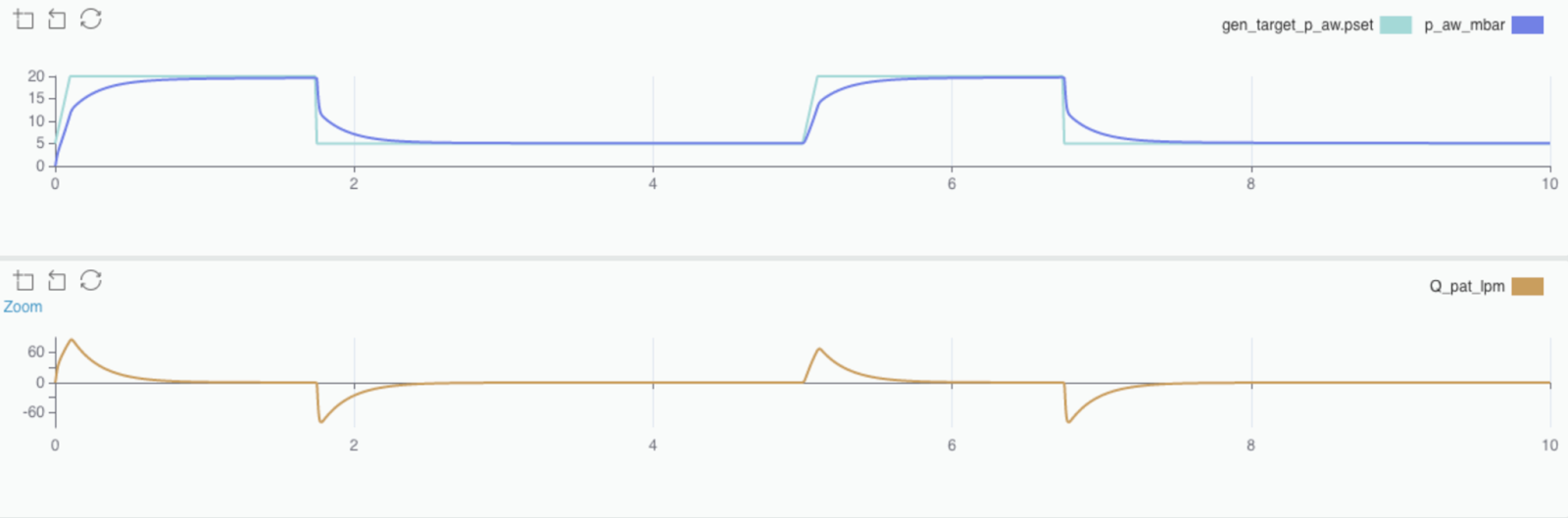Model with $k_i$ set to 0.4

While the pressure rise is still slow and unacceptable, the system does reach the target plateau pressure. The volumetric flow-rate response is smooth and does not exhibit any overshoots.

#### High gain: $k_i = 10$

With $k_i$ set to 10, the system output is shown below.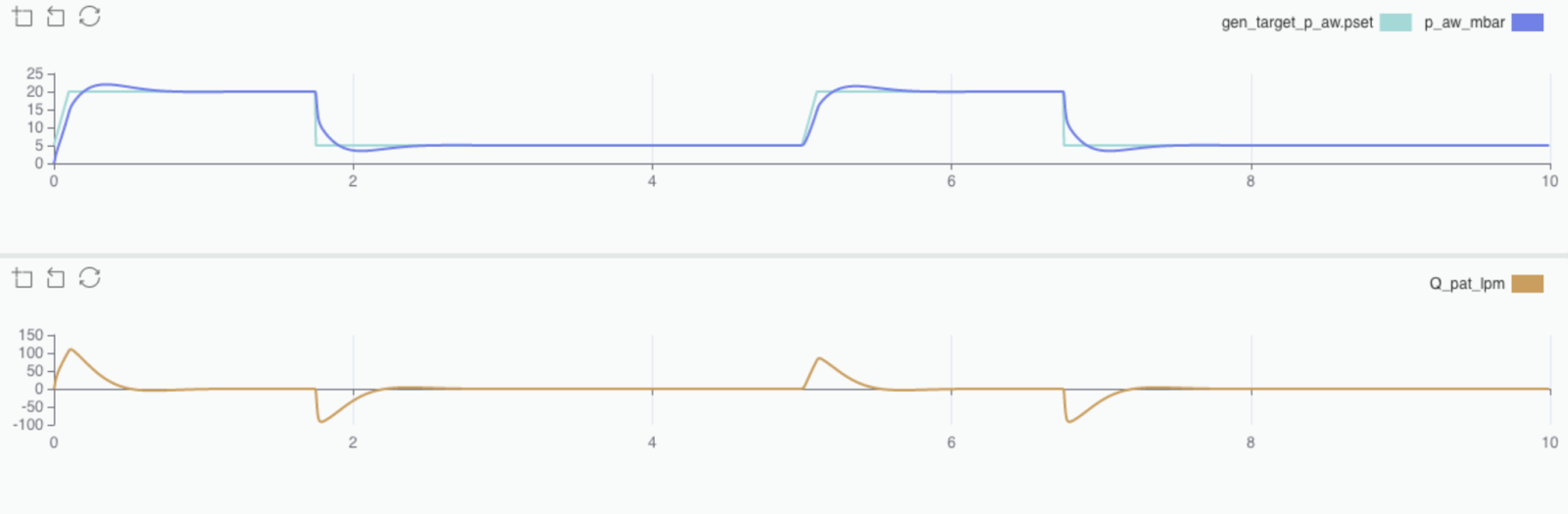Model with $k_i$ set to 10

A high gain results in a fast rise in the pressure (with acceptable pressure overshoot). However, the volumetric flow-rate exhibits overshoots. A zoomed-in view of the overshoot during the expiratory phase (our performance criteria) is shown below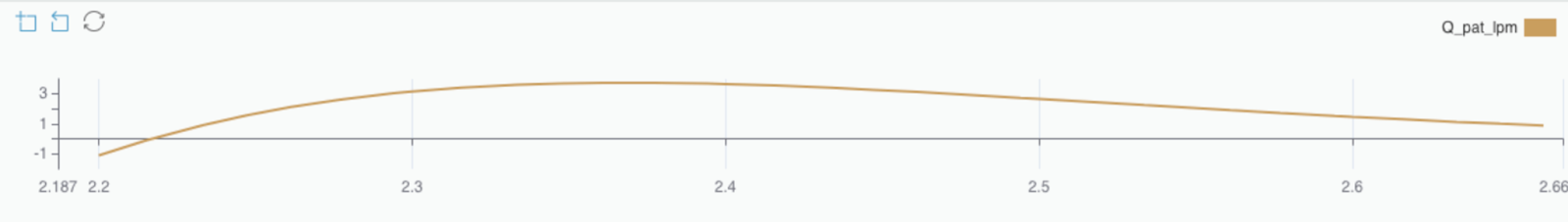$k_i$ set to 10 overshoot zoom

The peak overshoot is approximately 3.5 L/min, which violates our performance criteria.

From the above analysis we conclude that a low gain is desirable to limit overshoots in flow-rate, but a high gain is necessary to track the set pressure adequately and within desired rise time durations. A variable gain strategy can be utilized to combine the low and high gain controllers during the correct durations of the breathing cycle. This is described next.

### Variable gain control

A variable gain strategy can be designed based on the magnitude of the patient flow-rate $Q_\text{pat}$. The central idea is that when the magnitude of the flow-rate $Q_\text{pat}$ is high (say above a threshold $\delta$), a high gain controller can be used. On the contrary, when $Q_\text{pat}$ is low, a low gain controller, which minimises flow-rate oscillations, is preferable. This idea is depicted in the figure below, where a low gain is used by default, while a high gain is used when $|Q_\text{pat}|>\delta$.

The above nonlinear switch gain $\phi$ can be described with the following equation

$$\phi (Q_{\text{ pat}}) = \begin{cases} 0, & \text {if}~|Q_{\text{ pat}}| \leq \delta \\ \alpha, & \text {if}~|Q_{\text{ pat}} | > \delta \end{cases}, \tag{12}$$

where $\alpha$ is a high gain. The above switching gain can be applied to the error and passed on to the previously described low-gain controller with $k_i = 0.4$. A schematic of this is shown below.

In Collimator, we can design the switch nonlinear gain $\phi(Q_\text{pat})$ as a new sub-model. A schematic of this is shown below.

The above model implements equation (12), taking the inputs $Q_\text{pat}, \delta$, and $\alpha$ to compute $\phi$. The absolute value of $Q_\text{pat}$ is computed through the Abs block available within Collimator. Then a Comparator block is used to check if $|Q_\text{pat}|>\delta$. Finally, an If Then Else block is used to compute $\phi$.

The above sub-model can now be included in our system to implement the variable gain scheme. The complete schematic in Collimator is shown below.

In the research article , the authors suggest through experimentation and stability analysis that $\alpha = 18.5$ is the maximum gain that would be stable across a range of combinations of air connectors and patients (i.e. varying parameters of the hoses and lungs). To perform such stability analysis in Collimator please see this notebook. Lastly, $\delta$ does not affect the stability of the nonlinear controller. It can be chosen trial and error or by considering the response of a range of of hose-lung combinations. A good value for this $\delta = 6$ L/min . With these values, the system output is shown below.

The rise time and plateau pressure satisfy the performance criteria. Additionally, a zoomed-in plot of the flow-rate during the expiration phase shows that there are no noticeable overshoots.

Thus, the controller is acceptable and satisfies all the performance criteria. We encourage you to try this model in Collimator and assess system performance with varying parameters of the lungs and hoses. You can also assess the effect of changing $\delta$, $\alpha$, and the integral gain. Finally, for stability analysis of the variable gain controller, please see this notebook.

## Stability analysis of the variable gain controller

This notebook shows how to use Collimator for generating the transfer functions and plots for stability analysis of the variable gain controller. For a more thorough discussion on the controller and its stability, pleasee see the article below:

Hunnekens, Bram, Sjors Kamps, and Nathan Van De Wouw. "Variable-gain control for respiratory systems." IEEE Transactions on Control Systems Technology 28.1 (2018): 163-171.

import numpy as np
import matplotlib.pyplot as plt
import control
from math import pi



### The system parameters

Rlung = 5.0/1000
Clung = 20.0
Rleak = 60.0/1000
Rhose = 4.5/1000

zeta = 1.0
omega_n = 2.0*pi*30

ki = 0.4

iRlung = 1.0/Rlung
iClung = 1.0/Clung
iRleak= 1.0/Rleak
iRhose = 1.0/Rhose




### The state-space model for the hose and lung system

# Patient
# Eq (7) & (8) of the reference article 
A = -1.0*iRlung * iClung * (iRhose + iRleak) / (iRlung + iRhose + iRleak)
B = iRlung * iClung * (iRhose) / (iRlung + iRhose + iRleak)
C1 = iRlung / (iRlung + iRhose + iRleak)
C2 = -1.0*iRlung*(iRhose + iRleak) / (iRlung + iRhose + iRleak)
D1 = iRhose / (iRlung + iRhose + iRleak)
D2 = iRlung*D1

Ah = np.array([[A]])
Bh = np.array([[B]])
Ch = np.array([[C1],[C2]])
Dh = np.array([[D1],[D2]])



### The state-space representation of the blower

# Blower
# In the tutorial, we implemented this directly as a transfer function. The state-space representation is chosen to align with the reference article
# Eq (12) of the reference article  
Ab = np.array([[-2.0*zeta*omega_n, -1.0*omega_n**2],
[1.0, 0]])
Bb = np.array([,])
Cb = np.array([[0, omega_n**2]])



### Combine state-space model for the blower, hose and lung system

# Patient-Blower combined state-space model
# Eq (14) of the reference article  
Ap = np.block([[Ab, np.zeros((2,1))],
[Bh@Cb, Ah]])
Bp = np.block([[Bb],])
Cp = np.block([[Dh@Cb, Ch]])
Dp = np.zeros((2,1))



### Transfer function representation of the above state-space model

Ps = control.ss2tf(Ap,Bp,Cp,Dp)  # TF in Eq (15) of the reference article  
Pps = control.tf(Ps.num, Ps.den)  # TF corresponding to p_aw
Pqs = control.tf(Ps.num, Ps.den)  # TF corresponding to Q_pat



### Transfer function of the controller

Cs = control.tf(ki,[1,0])‍



### Transfer function of the Lur'e-type system associated with the variable gain controller

#### Nonlinear switch gain

$$\phi (Q_{\text{ pat}}) = \begin{cases} 0, & \text {if}~|Q_{\text{ pat}}| \leq \delta \\ \alpha, & \text {if}~|Q_{\text{ pat}} | > \delta \end{cases}.$$

#### State-space model

\begin{align*} \dot x=&Ax + B p_{\text{ set}} + B_{u} u \\[10pt] \begin{bmatrix} e \\ Q_{\text{ pat}} \end{bmatrix}=&C x + D p_{\text{ set}} \\[10pt] u=& = \phi (Q_{\text{ pat}}) e. \end{align*}

#### Transfer function

\begin{align*} e&=p_{\text{ set}} - p_{\text{ aw}} = \dfrac {P_{p}(s)C(s)}{1+P_{p}(s)C(s)} u + \dfrac {1-P_{p}(s)}{1+P_{p}(s)C(s)} p_{\text{ set}} \\[10pt] e&:= G_{eu}(s) u + G_{\text {ep}}(s) p_{\text{ set}}\\[20pt] \end{align*}

The article  shows that the switching nonlinear gain controller is input-to-state stable (ISS), if

(1) $G_{eu}(s)$ is Hurwitz,

(2) $\text{Re}[G_{eu}(j\omega)] > -1/\alpha$, and

(3) $1+G_{eu}(\infty) > 0$.

Geu = Pps*Cs/(1 + Pps*Cs)          # TF in Eq (20) of the reference article  
Gep = (1-Pps)/(1 + Pps*Cs)          # TF in Eq (20) of the reference article  



### Relevant plots

# Bode plot for Pps (combined system for hoses and lungs)
# Reproduce Fig 6 of the reference article  
mag, phase, omega = control.bode_plot(Pps, omega_limits=[1e-01, 1e03])



# Nyquist plot for Pps*Cs --> Reproduce Fig 13a of the reference article  
count = control.nyquist_plot(Pps*Cs)
ax = plt.gca()
ax.set_xlim(-1.5,0)


%%capture --no-display
# Nyquist plot for Geu --> Reproduce Fig 13b of the reference article  
count = control.nyquist_plot(Geu)
ax = plt.gca()
ax.set_xlim(-0.2,0)
ax.set_ylim(-0.2,0.2)

ax.vlines(-1/18.5, -0.2, 0.2, 'r')
tx = ax.text(-0.085,0.0,'$\\alpha = 18.5$', color='red')


# Bode plot of Geu --> Reproduce Fig 13c of the reference article  
mag, phase, omega= control.bode_plot(Geu)



## Considering a range of patient and hose combination

One can vary the hose and lung parameters in a wide range to find a suitable value of $\alpha$ that provides ISS stability across a range of hose-lung combinations. The code below generates a range of values for in the range $[\text{ref_value}/1.8, \text{ref_value}\times1.8]$ for $R_\text{lung}, C_\text{lung}, R_\text{hose}$, and $R_\text{leak}$, and generates the frequency responses.

def get_tfs(Rlung, Clung, Rhose, Rleak):
iRlung = 1.0/Rlung
iClung = 1.0/Clung
iRleak= 1.0/Rleak
iRhose = 1.0/Rhose

zeta = 1.0
omega_n = 2.0*pi*30

ki = 0.4

A = -1.0*iRlung * iClung * (iRhose + iRleak) / (iRlung + iRhose + iRleak)
B = iRlung * iClung * (iRhose) / (iRlung + iRhose + iRleak)
C1 = iRlung / (iRlung + iRhose + iRleak)
C2 = -1.0*iRlung*(iRhose + iRleak) / (iRlung + iRhose + iRleak)
D1 = iRhose / (iRlung + iRhose + iRleak)
D2 = iRlung*D1

Ah = np.array([[A]])
Bh = np.array([[B]])
Ch = np.array([[C1],[C2]])
Dh = np.array([[D1],[D2]])

Ab = np.array([[-2.0*zeta*omega_n, -1.0*omega_n**2],
[1.0, 0]])
Bb = np.array([,])
Cb = np.array([[0, omega_n**2]])

Ap = np.block([[Ab, np.zeros((2,1))],
[Bh@Cb, Ah]])
Bp = np.block([[Bb],])
Cp = np.block([[Dh@Cb, Ch]])
Dp = np.zeros((2,1))

Ps = control.ss2tf(Ap,Bp,Cp,Dp)
Pps = control.tf(Ps.num, Ps.den)
Pqs = control.tf(Ps.num, Ps.den)

Cs = control.tf(ki,[1,0])

Geu = Pps*Cs/(1 + Pps*Cs)

return Pps*Cs, Geu


Rlung_ref = 5.0/1000
Clung_ref = 20.0
Rleak_ref = 60.0/1000
Rhose_ref = 4.5/1000

npts = 3
factor = 1.8
Rlung_arr = np.linspace(Rlung_ref/factor, Rlung_ref*factor, npts)
Clung_arr = np.linspace(Rlung_ref/factor, Clung_ref*factor, npts)
Rhose_arr = np.linspace(Rlung_ref/factor, Rhose_ref*factor, npts)
Rleak_arr = np.linspace(Rlung_ref/factor, Rleak_ref*factor, npts)

Rlung_grid, Clung_grid, Rhose_grid, Rleak_grid = np.meshgrid(Rlung_arr, Clung_arr, Rhose_arr, Rleak_arr)


PpsCs_list = []
Geu_list = []
for i in range(npts):
for j in range(npts):
for k in range(npts):
for l in range(npts):
PpsCs, Geu = get_tfs(Rlung_grid[i,j,k,l],
Clung_grid[i,j,k,l],
Rhose_grid[i,j,k,l],
Rleak_grid[i,j,k,l])
PpsCs_list.append(PpsCs)
Geu_list.append(Geu)



# Nyquist plot for Pps*Cs --> Reproduce Fig 13a of the reference article  
count = control.nyquist_plot(PpsCs_list)
ax = plt.gca()
ax.set_xlim(-1.5,0)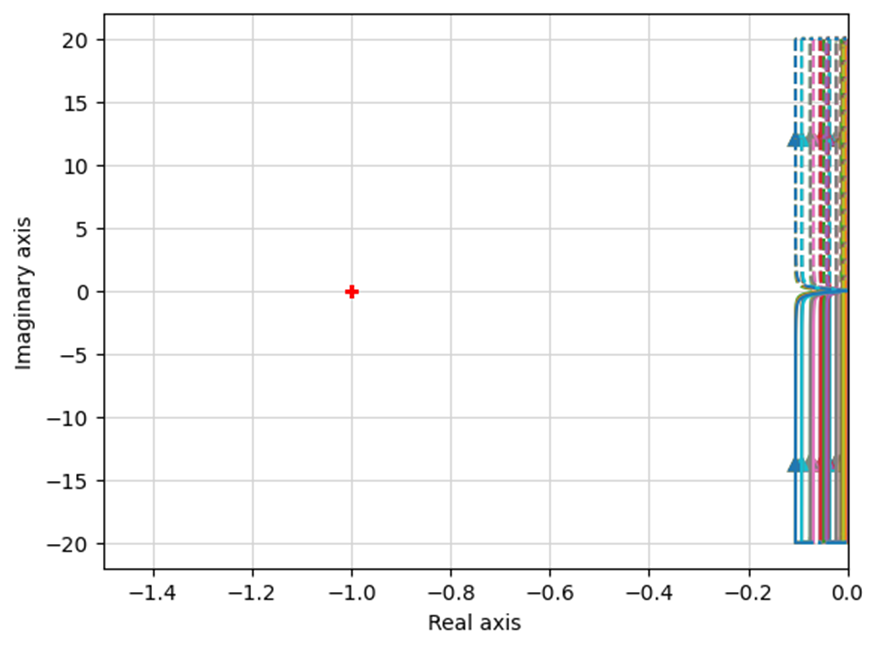$G_\text{eu}$ is Hurwitz

The above plot shows that $G_\text{eu}$ is Hurwitz. Thus, condition (1) is satisfied.

%%capture --no-display
# Nyquist plot for Geu --> Reproduce Fig 13b of the reference article  
count = control.nyquist_plot(Geu_list)
ax = plt.gca()
ax.set_xlim(-0.2,0)
ax.set_ylim(-0.2,0.2)

ax.vlines(-1/18.5, -0.2, 0.2, 'r')
tx = ax.text(-0.080,0.0,'$\\alpha = 18.5$', color='red')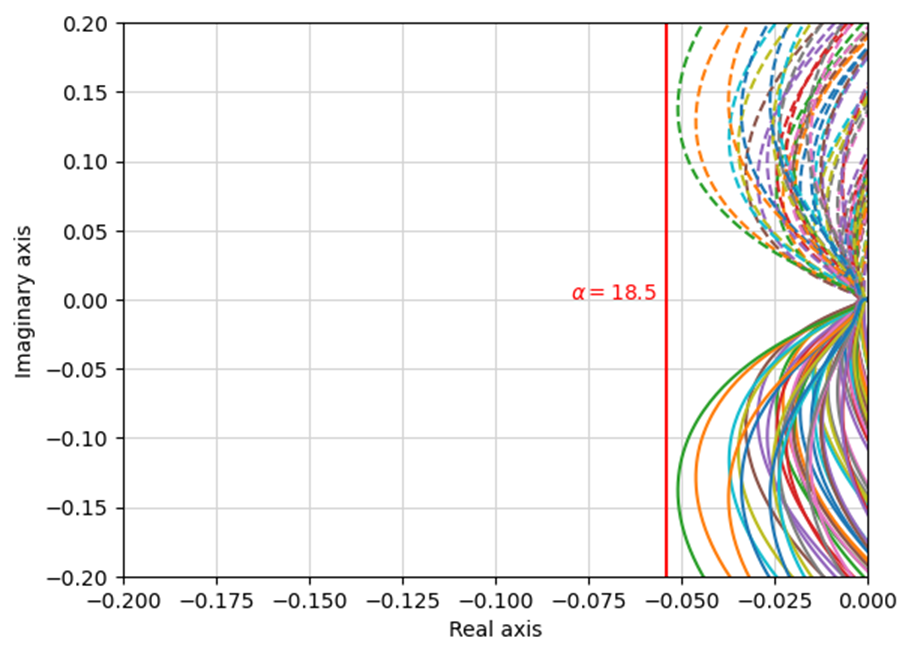$\text{Re}[G_{eu}(j\omega)] > -\dfrac{1}{\alpha}$ if $\alpha=18.5$

The above plot shows that $\text{Re}[G_{eu}(j\omega)] > -\dfrac{1}{\alpha}$ if $\alpha=18.5$ is chosen. Thus, condition (2) is satisfied within the range of patient-hose combinations considered and when $\alpha=18.5$.

# Bode plot of Geu --> Reproduce Fig 13c of the reference article  
mag, phase, omega= control.bode_plot(Geu_list)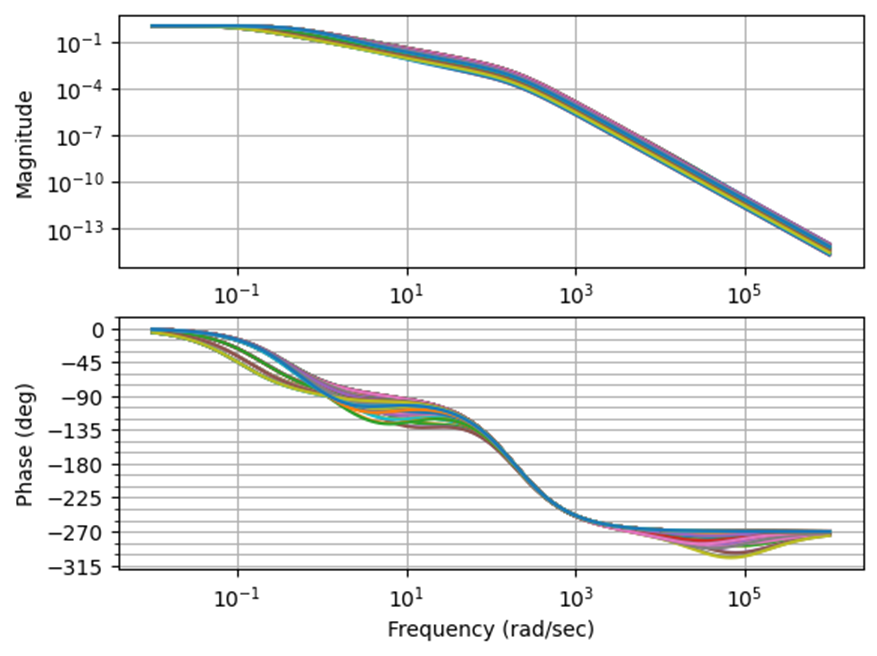$1+G_{eu}(\infty) > 0$

The above plot shows that $G_{eu}(\omega)$ has roll-off for high $\omega$ as the transfer function for the blower has a low-pass characteristic. Thus, $1+G_{eu}(\infty) > 0$ and condition (3) is satisfied.

## References

 Hunnekens, Bram, Sjors Kamps, and Nathan Van De Wouw. "Variable-gain control for respiratory systems." IEEE Transactions on Control Systems Technology 28